CBSE Sample Papers for Class 12 Computer Science Paper 1 are part of CBSE Sample Papers for Class 12 Computer Science. Here we have given CBSE Sample Papers for Class 12 Computer Science Paper 1.

## CBSE Sample Papers for Class 12 Computer Science Paper 1

 Board CBSE Class XII Subject Computer Science Sample Paper Set Paper 1 Category CBSE Sample Papers

Students who are going to appear for CBSE Class 12 Examinations are advised to practice the CBSE sample papers given here which is designed as per the latest Syllabus and marking scheme, as prescribed by the CBSE, is given here. Paper 1 of Solved CBSE Sample Paper for Class 12 Computer Science is given below with free PDF download Answers.

Time: 3 Hours
Maximum Marks: 70

General Instructions:

• All questions are compulsory within each Section.
• Programming Language in SECTION A : C++.

SECTION A

Question 1.
(a) Which of the following are not reserved keywords in C++?

WHILE, default, if, IF, do, INCLUDE, int, FLOAT

(b) Which C++ header file(s) will be essentially required to be included to run/execute the following C++ code?

void main( )
{
int Cno = 102; char CName[ ]= "Aayaan";
cout<<setw(5)<<Cno<<setw(25)<<CName<<endl;
}

(c) Rewrite the following program after removing the syntactical error(s), if any. Underline each correction.

#include
#include[stdio.h]
void main( )
{
struct movie
{
char movie_name;
char movie_type;
int ticket_cost = 100;
}
MOVIE;
gets(movie_name);
gets(movie_type);
}

(d) Find the output of the following C+ + program code:

void Change(int a = 25) .
{
for(int I = 10; I <= a; I+ = 4)
cout<<I<<" ";
cout< }
void Update(int &n)
{
n+ = 20:
Change(n);
}
void main()
{
int C = 15:
Update(C); '
Change( ):
cout<<"Number=''<<C<<endl:
}

(e) Find the output of the following program:

class TEACHER
{
int Tid, TeachCode, TeacherCount;
public:
TEACHER(int Thid=1)
{
Tid=Thid;
TeachCode=0;
TeacherCount=0;
}
void Subject(int S=20)
{
TeachCode++;
TeacherCount+=S;
}
void Status( )
{
cout<<Tid<<":"<<TeachCode<<”:”<<TeacherCount<<endl;
}
};
void main( )
{
TEACHER T(5), M:
T.Subject( );
M.Subject(50);
T.Status( ):
T.Subject(30);
M.Status( );
T. Status( );
}

(f) Observe the following program carefully, if the value of Num entered by the user is 5, choose the correct possible output(s) from the options from (i) to (iv). And also find the minimum and maximum value of Rndnum variable. Note: Assume all required header files are already being included. random(n) function will generate an integer between 0 to n-1.

void main( )
{
randomize( );
int Num, Rndnum;
cin>>Num;
Rndnum = random(Num)+5;
for(int N=1; N<=Rndnum; N++)
cout<<N<<" ";
}

Output
(i) 1 2 3 4
(ii) 1 2
(iii) 1 2 3 4 5 6 7 8 9
(iv) 1 2 3

Question 2.
(a) Explain the transitive nature of inheritance with an example.
(b) Answer the questions (i) and (ii) after going through the following class:

class Vehicle
{
int VehicleNo, Track;
public:
Vehicle( ); // Function1
Vehicle(int VH); // Function2
Vehicle(Vehicle &V); // Function3
void Allocated; // Function4
void Moved; // Function5
};
void main( )
{
Vehicle V;
:
:
}

(i) Out of the following, which option is correct for calling Function2?
Option 1 – Vehicle H(V);
Option 2 – Vehicle P(10);
(ii) Name the feature of object oriented programming which is illustrated by Function1, Function2 and Function3 combined together.
(c) Define the class Student with the following specifications:
Private members
sname 20 characters
eng, math, science float
total float
ctotal ( ) A function to calculate eng + math + science

Public members
Takedata( ) function to accept values for admno, sname, eng, math, science and invoke ctotal( ) to calculate total.
Sbowdata( ) function to display all the data members on the screen.
(d) Answer the questions (i) to (iv) based on the following code:

class MANAGER
{
int Mgr_no;
char Mgr_Name;
protected:
char Dept;
public:
MANAGER( );
void Mentry( );
void Mdisplay( );
};
class EMPLOYEE
{
int emp_no;
char emp_name;
protected:
float salary;
public: .
EMPLOYEE( );
void Eentry( );
void Edisplay( );
};
class COMPANY:private MANAGER, public EMPLOYEE
{
char cmp_no;
char estb_date:
public:
char description;
COMPANY( );
void Centry( );
void Cdetail( ):
};

(i) Which type of inheritance is shown in the above example?
(ii) Write the names of data members which are accessible from objects belonging to class COMPANY.
(iii) Write the names of all the member functions which are accessible from objects belonging to class COMPANY.
(iv) Write the names of all the data members which are accessible from member functions of class EMPLOYEE.

Question 3.
(a) Write a function in C++ which accepts an integer array and its size as arguments and replaces elements having odd values with thrice its value and elements having even values with twice its value.
e.g. If an array of five elements initially contains the elements as 3, 4, 5, 16, 9
Then, the function should rearrange the content of the array as 9, 8, 15, 32, 27
(b) An array P  is stored in the memory along the column with each of the element occupying 2 bytes. Find out the memory location for the elements P , if the base address of the array is 6800.
(c) Given the necessary declaration of linked implemented queue containing players information (as defined in the following definition of Node). Also, write a user defined function in C++ to delete one player’s information from the queue.

struct Node
{
int PlayerNo;
char PIayerName;
};
class QUEUE( )
{
Node *front, *rear;
public:
QUEUE( )
{
front=rear=Null;
}
void Insert_Node( );
void Delete_Node( );
~QUEUE( );
};

(d) Change the following infix expression into postfix expression:
((A + B) * C + D/E – F)
(e) Write a function in C++ to find the sum of both left and right diagonal elements from a two dimensional array (matrix).
e.g. If array contains
4 3 5
6 7 4
5 6 7
The output will be
Sum of Diagonal 1 : 18
Sum of Diagonal 2 : 17

Question 4.
(a) Find the output of the following C++ code considering that the binary file STU.DAT exists on the hard disk with a data of 50 students.

class Student
{
int Rno;
char Sname;
public:
int count( );
};
void main( )
{
fstream f;
f.open("STU.DAT", ios::binary|ios::in);
Student S;
int a=1;
while(a<2)
{
a++ ;
}
int Position=f.tellg( )/sizeof(S);
cout<<"\n Present Student Record:"<<Position<<endl;
f.close( );
}

(b) Write a function in C++ to print the count of the word “is” as an independent word in a text file “DIALOGUE.TXT”. e.g. If the content of the file DIALOGUE.TXT is This is his book. Is this book good? Then the output of the program should be 2.
(c) Assuming a binary file “JOKES.DAT” is containing objects belonging to a class JOKE (as defined below). Write a user-defined function in C++ to add more objects belonging to class JOKE at the bottom of it.

class JOKE,
{
int Jokeid; // Joke identification number
char Type; // Joke type
char Jokedesc; // Joke description
public;
void NewJokeEntry( )
{
cin>>Jokeid;gets(Type);
gets(Jokedesc);
}
void ShowJoke( )
{
cout<<Jokeid<<": "<<Type<<endl<<Jokedesc<<endl;
}
};

SECTION B

Question 5.
(a) Differentiate between primary key and candidate key of a table with the help of an example.
(b) Write SQL queries for (i) to (iv) and find outputs for SQL queries (v) to (viii). Which are based on the tables?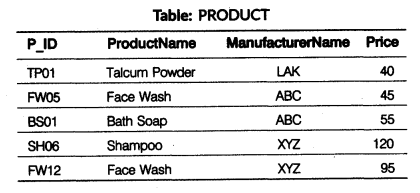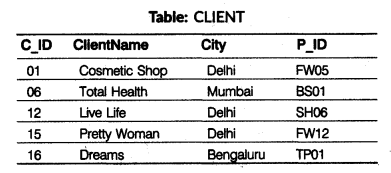(i) To display the details of all the clients except those whose city is Delhi.
(ii) To display the ClientName, City from table CLIENT and ProductName and Price from table PRODUCT, with their corresponding matching P_ID.
(iii) To display names of those clients whose city is Delhi and has ‘e’ as third letter in their name.
(iv) To display product names and price in decreasing order of price.

(v) SELECT DISTINCT City FROM CLIENT;
(vi) SELECT ManufacturerName, MAX(Price), MIN(Price), COUNT(*)
FROM PRODUCT GROUP BY ManufacturerName;
(vii) SELECT ClientName, ProductName FROM CLIENT, PRODUCT
WHERE CLIENT.P_ID = PRODUCT.P_ID;
(viii) SELECT ProductName, Price, ClientName, City FROM PRODUCT, CLIENT
WHERE PRODUCT.P_ID=CLIENT.P_ID AND Price<90;

Question 6.
(a) Verify the following using Boolean laws:
$$\bar { X } Y+X\bar { Y } +\bar { X } \bar { Y } =\left( \bar { X } +\bar { Y } \right)$$
(b) Write the equivalent Boolean expression for the following logic circuit: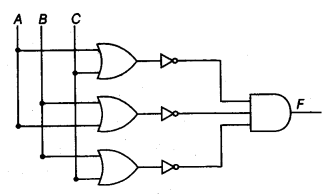(c) Given the following truth table, derive a Sum of Product (SOP) form of Boolean expression from it: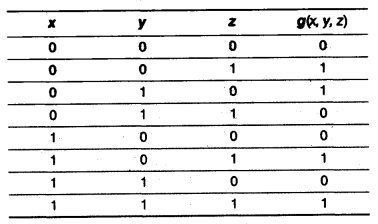(d) If F(A, B, C, D) = π(0, 2, 4, 5, 7, 8, 10, 12, 13, 15), obtain the simplified form using K-map.

Question 7.
(a) Which network device is used to amplify the feeble signals when they are transported over a long distance? Also, write its function.
(b) Give two major reasons to have network security.
(c) How does node failure cause network failure in a ring topology?
(d) What is the purpose of using a Web browser? Name any one commonly used Web browser.
(e) Knowledge Supplement Organisation (KSO) has set-up its new center at Bengaluru for its office and Web-based activities. It has 4 blocks of buildings as shown in the diagram below: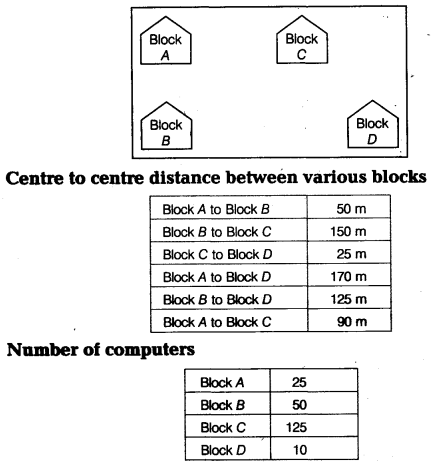(i) Suggest a cable layout of connections among the blocks.
(ii) Suggest the most suitable place, i.e. Block to house the server of this organization with a suitable reason.

(iii) Which type of network out of the following is formed be a connection the computers of these three blocks?
I. LAN
II. MAN
III. WAN

(iv) Which wireless channel out of the following should be opted by (KSO) to connect to people from all over the world?
I. Infrared
II. Microwave
III. Satellite

(f) Categorize the following client side and server side script category:
(i) PHP
(ii) VB Script
(iii) ASP
(iv) JavaScript

(g) List the characteristics of a computer virus.

(a) WHILE, IF, INCLUDE, FLOAT are not reserved keywords in C ++.

(b) <iomanip.> → setw( )
<iostraem.h> → cout( )

(c) #include
#include
void main( )
{
struct movie
{
char movie_name;
char movie_type;
int ticket cost;
} MOVIE;
MOVIE.ticket cost=100:
qets(MOVIE, movie name):
cin>>MOVIE, movie type:
}

(d) Output
10 14 18 22 26 30 34
10 14 18 22
Number = 35
(e) Output
5 : 1 : 20
1 : 1 : 50
5 : 2 : 50
(f) The possible output is (iii) 1 2 3 4 5 6 7 8 9
The minimum value of Rndnum = 5
The maximum value of Rndnum = 9

(a) Inheritance is transitive, i.e. if a class B inherits properties of another class A, then all subclasses of B will automatically inherit the properties of class A. This property is called transitive nature of inheritance,
e.g.

class A .
{ int a;
public:
void setA( )
{
cin>>a;
}
};
class B:public A
{
int b;
public:
void setB( )
{
cin>>b;
}
};
class C:public B
{
int c;
public:
void setC( )
{
cin>>c;
}
};

The above example show the transitive nature of inheritance,
(b) (i) Option 2-Vehicle P(10); is correct

(c) class Student
{
char sname;
float eng, math, science;
float total;
ctotal( )
{
float sum = eng + math + science;
return sum;
}
public:
void Takedata( )
{
cout<<"Enter marks in English\n"; cin>>eng;
cout<<"Enter marks in Maths\n";cin>>math;
cout<<"Enter marks in Science\n";cin>>science;
ctotal( );
}
void Showdata( )
cout<<"Name\n"<<sname<<endl;
cout<<"Marks in Engl ish\n"<<eng<<endl;
cout<<"Marks in Maths\n"<<math< cout<<"Marks in Science\n"<<science<<endl;
cout<<"Total Marks\n"<<total;
}
};

(d) (i) Multiple Inheritance
(ii) description
(iii) Centry( ), Cdetail( ), Eentry( ), Edisplay( )
(iv) emp_no, emp_name, salary

(a) void replace(int *arr, int Size)
{
int i;
for(i=0; i<Size; i++)
if(arr[i]%2 == 0)
arr[i]=arr[i]*2;
else
arr[i]=arr[i]*3;
for(i=0; i<Size; i++)
cout<<arr[i]<<" ";
}

R = 50
Size of element W=2 bytes
I = 10, J = 20, Ir =0, Ic = 0
P[I][J] = B + W*[(I-Ir) + R*(J-Ic)]
P  =6800 + 2 * [(10 – 0) + 50 * (20 – 0)]
= 6800 + 2 * [10+1000]
= 6800+ 2 *
= 6800 + 2020 = 8820

(c) void Delete_Node( )
{
Node *P;
if(front==Null)
cout<<"Underflow":
else if (front==rear)
{
P=front;
cout<<"Deleted Node information is";
cout<PlayerNo;
puts(P->playerName);
front=rear=Null;
delete P;
}
else
{
P=front;
cout<<"Deleted Node information is";
cout<PlayerNo;
puts(p->PlayerName);
delete P;
}
}

(d) Given, expression as ((A + B)* C + D/E – F)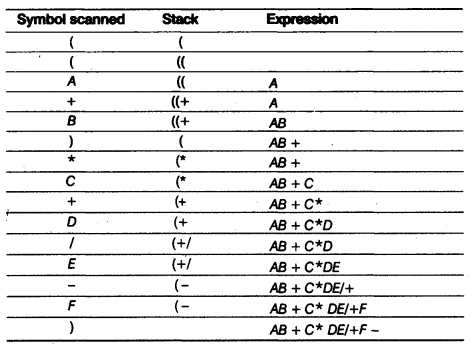Hence, the postfix expression is AB + C * D/E-F

(e) void DiagSum(int AC[ ]C5], int N) //A[ ][ ] is a matrix of size m x n and m = n
{
int SumD1=0, SumD2=0;
for(int I=0; I<N; I++)
{
SumD1 =A[I][I]; SumD2+=A[N-I-1][I];
}
cout<<"Sum of Diagonal 1 : "<<SumD1<<endl;
cout<<"Sum of Diagonal 2 : "<<SumD2<<endl;
}

(a) Present Student Record: 1

(b) void COUNT( )
{
fstream File;
Fi1e.open("DIALOGUE.TXT", ios::in);
char word; int c=0;
File>>word;
while(!File.eof(.))
{
for(int i=0; i<strlen(word); i++)
word[i] = tolower(word[i]);
if(strcmp(word,"is")=0) C++;
File>>word;
}
cout<<"count of is in file"<<c<<endl;
File.close( );
}

{
char ans = "y";
fstream file;
file.open("JOKES.DAT", ios::app | ios::binary);
ans = getchar( );
while(ans == ’y’)
{
JOKE J1;
J1.NewJokeEntry( );
file.write((char *)&J1, sizeof(J1));
ans = getchar( );
}
file.close( );
}

(a) A candidate key can be any column or a combination of columns that can qualify as unique key in the database. There can be multiple candidate keys in one table. While the Primary key is a column that uniquely identifies a record. A relation can have only one primary key.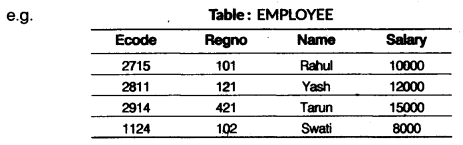In the EMPLOYEE table, Encode and Regno both can identify uniquely. So, both are candidate keys. Here, we have created Encode as a primary key.

(b) (i) SELECT * FROM CLIENT WHERE City NOT IN (’Del hi');
(ii) SELECT ClientName, City, ProductName, Price FROM CLIENT, PRODUCT
WHERE CLIENT.P_ID = PRODUCT.P_ID;
(iii) SELECT ClientName FROM CLIENT
WHERE City='Delhi' AND ClientName LIKE"__e%";
(iv) SELECT ProductName, Price FROM PRODUCT ORDER BY Price DESC;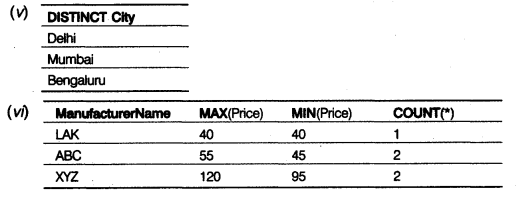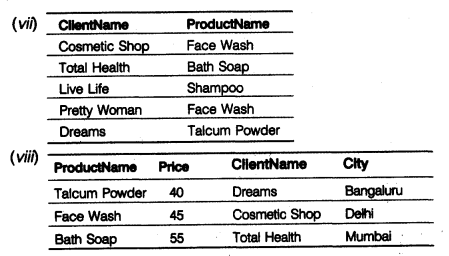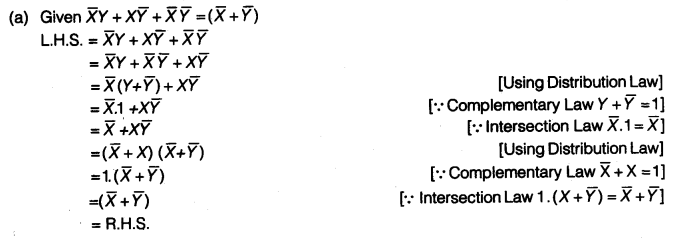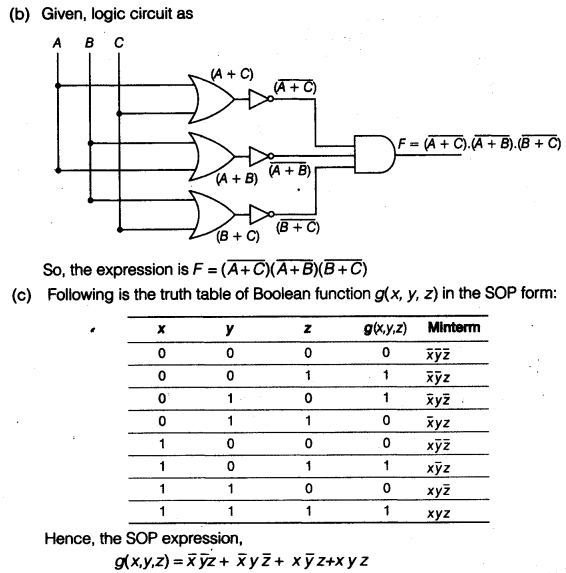(a) Repeater: The basic function of a repeater is to amplify the incoming signal and retransmit it, to the other device.
(b) Two major reasons to have network security are as follows:
(i) Security keeps information secure from unauthorized users.
(ii) Authentication determining that the user. is. authorized before sharing sensitive information with or entering into a business deal.
(c) The transmission of data in a ring goes through every connected node on the ring before returning to the sender. If one node fails to pass data through itself, the entire network has failed and no traffic can flow until the defective node has been removed from the rang.
(d) The Web browser fetches the page requested, interprets” the text and formatting commands that it contains and displays the page properly formatted on the screen, e.g. Mozilla Firefox.
(e) (i) Cable Layout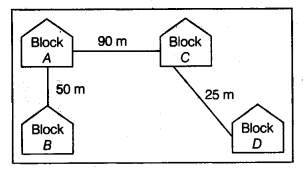(ii) The most suitable place/block to house the server of this organization would be Block C, as this block contains the maximum number of computers, thus decreasing the cabling cost for most of the computers as well as increasing the efficiency of the maximum computers in the network.
(iii) I. LAN because its coverage area is up to 5 km.
(iv) III. Satellite because its coverage area is in all over the world.
(f) Client-side script
(iii) VB Script
(iv) JavaScript
Server-side script
(i) PHP
(ii) ASP
(g) The following are the characteristics of a computer virus:
(i) it is able to replicate.
(ii) It requires a host program as a carrier.
(iii) It is activated by external action.
(iv) Its replication ability is limited to the system.

We hope the CBSE Sample Papers for Class 12 Computer Science Paper 1 help you. If you have any query regarding CBSE Sample Papers for Class 12 Computer Science Paper 1, drop a comment below and we will get back to you at the earliest.